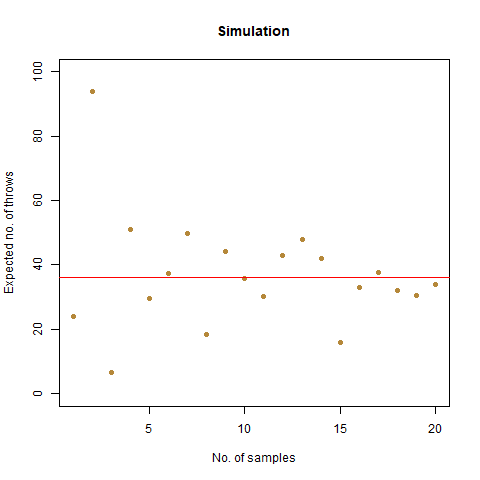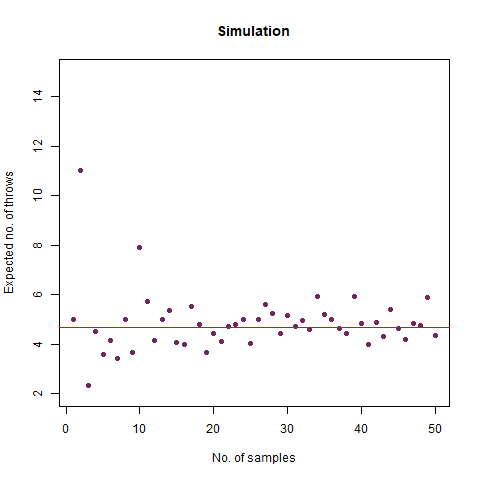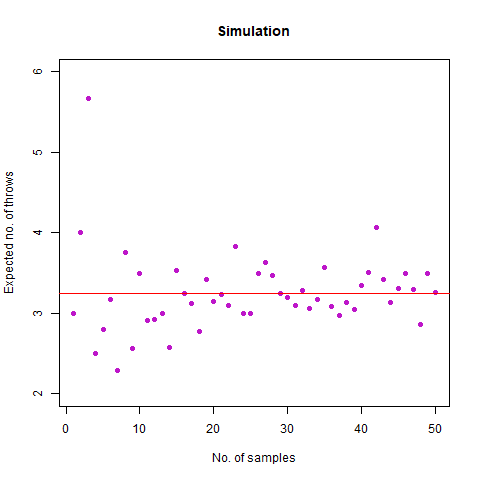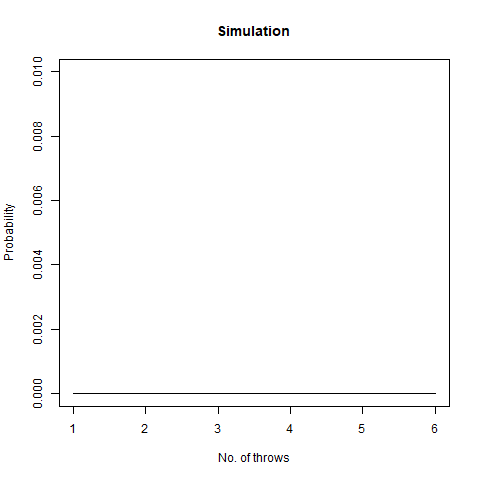Want to share your content on R-bloggers? click here if you have a blog, or here if you don't.

It was when I and one of my friends, Ahel, were playing a game of Ludo, that an idea struck both of our heads. Being final year undergraduate students of Statistics, both of us pondered upon the question, what if we can do some verification for some questions on dice. This brief spark in our brains led us to properly formulate the questions, think and write the solutions using mathematical concepts, and finally simulate them. Note that we did all the simulations using the R programming language. We also included the `animation` package in R for some visualisations of our graph.

So here goes our questions:

### On average, how many times must a 6-sided die be rolled until a 6 turns up?

This seems like an easy one, right? It tells us, that if we are to roll a normal 6-sided die, when can we expect the face showing 6 to turn up. We create a function to do this simulation:

We then do a Monte Carlo simulation, to get as close to our theoretical answer as possible:

The result we got was `6.018` , which, almost coincides with the theoretical value of `6` . We further create a graph that helps us see the convergence more clearly.The graph of the expected number of throws vs sample numbers. We notice that as we take a larger sample size, the expected number of throws converges to 6 which is the exact number of throws shown mathematically.

### On average, how many times must a 6-sided die be rolled until a 6 turns up twice a row?

We can think of this as an extension of the previous problem. However, in this case, we will stop after two successive rolls result in two 6s. Thus, if we roll a 6, we roll again and then we will get any one of the two results:

• We get a 6 again. If this happens, we stop
• We get anything other than a 6. Then we continue rolling.

We can use a function to simulate, which is a bit different from the last one:

We then do a Monte Carlo simulation, to get as close to our theoretical answer as possible:

The result that was expected (as shown mathematically) is `42` . From the simulation, we got the result as `42.32468` , which is almost as close as our theoretical observation. The below graph summarises the above statements:The graph of the expected number of throws vs sample numbers. We notice that as we take a larger sample size, the expected number of throws converges to 42 which is the exact number of throws shown mathematically.

### On average, how many times must a 6-sided die be rolled until the sequence 65 appears (that is a 6 followed by a 5)?

What are the possible cases that we can run into, after throwing a 6?

• We roll again, and we get a 6. This is a redundant case and we move again.
• We roll again, and we get a 5. We stop if this happens.
• We roll again, and we get something else. We continue rolling.
• We roll again, and we get a 6. This is a redundant case and we move again.
• We roll again, and we get a 5. We stop if this happens.
• We roll again, and we get something else. We continue rolling.

We then do a Monte Carlo simulation, to get as close to our theoretical answer as possible:

We expected the number of throws to be `36` . Upon doing simulation, we found our result as `36.07392` , which is almost accurate. We have the following animation which summarises the fact:The graph of the expected number of throws vs sample numbers. We notice that as we take a larger sample size, the expected number of throws converges to 36 which is the exact number of throws shown mathematically.

#### Note:

The number of throws, in this case, is smaller than in the previous case, though both appear to be almost the same. The reason is intuitive in the fact that after rolling a 6, we can find three cases for this question, of which one is redundant, but not to be ignored, whereas, for the previous one, we only find two possible scenarios.

### On average, how many times must a 6-sided die be rolled until two rolls in a row differ by 1 (such as a 2 followed by a 1 or 3, or a 6 followed by a 5)?

This is an interesting one. Suppose we roll a 4. Then three cases may happen:

• We roll a 3. In that case, we stop.
• We roll a 5. In that case, we stop.
• We roll anything else. Here, we continue rolling.

Consider the following function for our work:

We then do a Monte Carlo simulation, to get as close to our theoretical answer as possible:

The result of the simulation was `4.69556` which is much closer to the theoretically expected value of `4.68` . We again provide a graph, which describes the convergence adequately:The graph of the expected number of throws vs sample numbers. We notice that as we take a larger sample size, the expected number of throws converges to 4.68 which is the exact number of throws shown mathematically.

#### Bonus Question: What if we roll until two rolls in a row differ by no more than 1 (so we stop at a repeated roll, too)?

This question differs from its other part in the sense that here, successive rolls can be equal also for the experiment to stop. With just a minute tweak in the previous function, we can derive the new function for simulating this problem:

We then do a Monte Carlo simulation, to get as close to our theoretical answer as possible:

We expected the value to be close to our mathematical value of `3.278` . From the simulation, we obtained the result as `3.292` . Further, we create the following graph:The graph of the expected number of throws vs sample numbers. We notice that as we take a larger sample size, the expected number of throws converges to 3.27 which is the exact number of throws shown mathematically.

### We roll a 6-sided die n times. What is the probability that all faces have appeared?

Intuitively, as the number of throws is increased, i.e. `n` increases, the probability that all the faces have appeared reaches `1` . Fixing a particular value of `n` , the mathematical probability which we got is as follows:

Now suppose we would like to simulate this experiment. Consider the following function for the work:

We vary our `n` from 1 to 100 and find that after `n=60` , the probability becomes `1` almost surely. This is depicted in the following graph:The graph of the probability of getting all faces vs the number of throws. We notice that as we number of throws, this probability converges to 1.

### We roll a 6-sided die n times. What is the probability that all faces have appeared in order in six consecutive throws?

In short, this question asks what is the probability that among all the throws, sequence 1,2,3,4,5,6 appears. We once again create a function for our task:

The following graph demonstrates that as the number of throws increases, this probability increases:The graph of the probability of getting all faces vs the number of throws. We notice that as we number of throws, this probability increases.

Taking our `n` as `300000` , we find that this probability becomes around `0.99` . This implies that the probability converges to 1 as `n` increases.

### Person A rolls n dice and person B rolls m dice. What is the probability that they have a common face showing up?

This question asks us that if person A rolled a 2, then what is the probability that person B also rolled a 2 among all the dice throws. This is a pretty straightforward question. Intuitively, we can observe, that the value of `n` and `m` increases (even a slight bit as >12), the corresponding probability reaches 1. We once again take the help of a function for our purpose:

We then create a matrix structure which gives us the probability for the values of `m` and `n` :

The underlying graph confirms this intuition:We find from this graph that as m and n increases (even >12), the value of this probability reaches 1

### On average, how many times must a pair of 6-sided dice be rolled until all sides appear at least once?

Suppose, we have a die, and we throw it. Then this question asks us to find out the average number of such throws required such that all the faces appear at least once. Now simulating this experiment can be done in a pretty interesting way with the help of a Markov Chain. However, for the sake of simplicity, consider the following function for our use case:

The mathematical value that we got after calculating is around 7.6 which, when rounded off becomes 8. We then proceed to do a Monte Carlo simulation of our experiment:

Here, we find that the value is 7.5, which again rounds off to 8. That’s not the end of it. We further create a plot which can sharpen our views regarding this experiment:

Further, we find that the probability that our required rolls will be more than 24 is almost negligible (at around 7e-04).

### Suppose we can roll a 6-sided die up to n times. At any time we can stop, and that roll becomes our “score”. Our goal is to get the highest possible score, on average. How should we decide when to stop?

This is a particularly vexing problem. We note that our stopping condition is when we will get a number smaller than the average at the nth throw. Consider the following function for this problem:

We proceed to do a Monte Carlo Simulation:

We thus create a stopping rule and draw the following conclusion regarding the highest possible score on an average:

• If n=1, we choose our score as 3

• If 1

• If n>3, we choose our score as 5

Finally, we draw a graph of our findings:

### Suppose we roll a fair dice 10 times. What is the probability that the sequence of rolls is non-decreasing?

Suppose we get a sequence of faces as such: 1,1,2,3,2,2,4,5,6,6. We can clearly see that this is not at all a non-decreasing sequence. However, if we get a sequence of faces as like: 1,1,1,1,2,2,4,5,6,6, we can see that this is a non-decreasing sequence. Our question asks us to find the probability of getting a sequence like the second type. We again build a function as:

We expect our answer to be around 4.96e-05. We continue to do the Monte Carlo simulation:

The result that we get after simulating is 5.1e-05 which is pretty close to our value. Finally, we create a graph:

We thus come to the end of our little discussion here. Do let us know how you feel about this whole thing. Also, feel free to connect with us on LinkedIn:

Debartha Paul

Ahel Kundu

You might also want to check out the GitHub repository for this project here: Dice Simulations

Some problems related to Dice was first posted on November 19, 2022 at 3:08 pm.